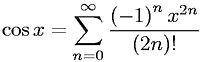Equations > Calculus > Sequences and Series > Cosine Definition as an Infinite Series

### Cosine Definition as an Infinite SeriesLatex Code:

MathML Code:

 $\mathrm{cos}x=\sum _{n=0}^{\infty }\frac{\left(-1\right)}^{n}{x}^{2n}\left(2n\right)!$

MathType 5.0: